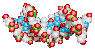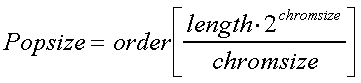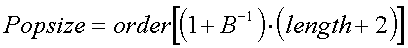# Genetic Algorithm (GA) Parameter Settings

The efficiency of a GA is greatly dependent on its tuning parameters. Your choices are to either use one of several 'standard' parameter settings or to calculate your own settings for your specific problem.

## Standard Parameter Settings

There are several recommended settings:### Dejong Settings (From [DeJong and Spears, 1990]):

Dejong's settings are the de facto standard for most GAs. Dejong has shown that this combination of parameters works better than many other parameter combinations for function optimization.

• Population size 50
• Number of generations 1,000
• Crossover type= typically two point
• Crossover rate of 0.6
• Mutation types= bit flip
• Mutation rate of 0.001

Notes:

The crossover method is assumed to be one or two point crossover. For more disruptive methods (such as uniform crossover), use a lower crossover rate (say 0.50).
The mutation rate given above is *per bit*, whereas in many public domain codes, the mutation rate is input as a *per chromosome* probability. Make sure your mutation rates aren't too low!

REFERENCE:

DeJong, K.A. and Spears, W.M. "An Analysis of the Interacting Roles of Population Size and Crossover in Genetic Algorithms," Proc. First Workshop Parallel Problem Solving from Nature, Springer-Verlag, Berlin, 1990. pp. 38-47.### Grefenstette Settings (From [Grefenstette, 1986]):

This is generally the second most popular set of parameter settings. It is typically used when the computational expense of figuring the objective function forces you to have a smaller population.

• Population size 30
• Number of generations not specified
• Crossover type= typically two point
• Crossover rate of 0.9
• Mutation types= bit flip
• Mutation rate of 0.01

Notes:

The crossover method is again assumed to be one or two point crossover and the mutation rate is also *per bit*.

REFERENCE:

Grefenstette, J.J. "Optimization of Control Parameters for Genetic Algorithms," IEEE Trans. Systems, Man, and Cybernetics, Vol. SMC-16, No. 1, Jan./Feb. 1986, pp. 122-128.### MicroGA Settings From David L. Carroll

These settings are not widely employed currently, but those who have used them to optimize functions report as much as four-fold reductions in the number of evaluations required to reach given levels of performance.

• Population Size = 5 (see below)
• Number of generations = 100
• Crossover type= uniform
• Crossover probability= 0.5
• Mutation types= jump and creep
• Mutation probabilities= 0.02 and 0.04 (see below)

Notes:

David's code continously restarts with random chromosomes when convergence is dectected, so these parameters may not work well for other codes.### Rough Comparison

I tried these sets of parameters in Sugal, a nice GA with a GUI interface for MS-Windows. The problem is a Dejong error function, the goal is to minimize the error. Below is a screen print showing how quickly each parameter setting found the minimum. I've in no way tried to be exhaustive about this test case, and you should be suspicious that Dejong's parameters do best on a Dejong problem. Its simply meant to give you some idea of the relative strengths of the different settings.

## Customizing Parameter Settings### Population Size

Some problems have very large solution spaces (i.e. many variables, with a large range of permissible values for those variables). In these cases, a population of 100 individuals is probably not enough because it simply doesn't represent a large enough sample of the solution space. The following rules of thumb will help determine how big a population you will need.

The following crude population scaling law is based on [Goldberg & Deb, 1992] courtesy of David L. Carroll

•for binary coding

where

length = number of binary bits in each individual
chromsize = the average size of the schema of interest (effectively the average number of bits per chromosome, i.e. length/Number of parameters encoded by one individual), rounded to the nearest integer

David notes that this scaling law is usually overkill, i.e. you can most likely get by with populations at least twice as small.

Drawing on the statistical calculations of another discpline, SunTzu1@aol.com provides another possibile method for calculating the population size:

In the field of cluster analysis there is a relationship between the quality of the estimate of the cluster statistics, the number of samples in the cluster, and the number of parameters defining a cluster member. It may be possible to view each chromosome as a vector and, in order to prevent biases from creeping into the solutions, the population (number of samples) must increase as the vector size increases. This relationship is:

•for binary coding

where

length = number of binary bits in each individual
B = a measure of the confidence interval for the statistical estimate (typically 0.05 or 0.1)### Number of Generations

When do you stop a GA? The following observations are from Jeffrey Horn

In a basic ("simple") GA with selection, crossover, and low (or no) mutation), the population should indeed converge in "just a few" generations (e.g., 30 to 50 gens with a population of size 1000). Goldberg and Deb (1991) indicated that pure selection convergence times are O(log N) generations, where N is population size. This is indeed what I observe. So GA search is over fairly quickly. This can be turned into a strength of the GA by using larger population sizes (Goldberg, Deb, & Clark, 1992) and/or restarting the GA with a new random initial population upon detection of population convergence (Goldberg, Kargupta, Horn, & Cantu-Paz, 1995).
By encoding some linkage knowledge into the chromosome, "mixing" of good building blocks can be expected to take place before convergence (given adequate population sizes also) (Thierens & Goldberg, 1993).
So I believe it is important to detect near-uniformity of the population and terminate the GA, before wasting function evaluations on an inefficient, mutation-based search. Of course, if you maintain useful diversity in your populations using a "restorative force" to balance convergence, such as a niching method (Horn, 1993; Mahfoud, 1995), then all bets are off; there is no (practical) convergence to be detected. Apparently, useful search continues during some kind of noisy, but stable and diverse, steady state.
There are a number of estimates of population convergence. I like the "bit-wise average convergence measure" we used in some of the experiments in (Goldberg, Kargupta, Horn, & Cantu-Paz, 1995). We measured the convergence at each loci (i.e., the percentage of the population with a single allele at that locus) and averaged over all loci. When this average exceeds some threshold, say 90%, we call it quits. I do not think the performance of the GA, especially w.r.t solution quality, is much affected by the EXACT method/threshold of convergence measure.

REFERENCES:

Goldberg, and Deb, (1991). A comparative analysis of selection schemes used in GAs. In G. Rawlins (Ed.), Foundations of GAs (FOGA). San Mateo, CA: Morgan Kaufmann, 69--93.
Goldberg, D.E., Deb, K., & Clark, J.H. (1992). Genetic algorithms, noise, and the sizing of populations. Complex Systems, 6. 333-362.
Goldberg, D. E., Kargupta, H., Horn, J., & Cantu-Paz, E. (1995). Critical deme size for serial and parallel genetic algorithms. IlliGAL Report 95002 (The Illinois GA Lab, University of Illinois). 24 pp.
Thierens, D., & Goldberg, D.E. (1993). Mixing in genetic algorithms. In S. Forrest (Ed.), Proceedings of the Fifth International Conference on Genetic Algorithms (ICGA 5). San Mateo, CA: Morgan Kaufmann, 38-45.
Horn, J. (1993). Finite Markov chain analysis of genetic algorithms with niching. In ICGA 5, 110-117.
Mahfoud, S. W. (1995). Niching methods for genetic algorithms . 251 pp. (a dissertation from the University of Illinois). (also IlliGAL Report No. 95001)

The above publications are available from IlliGAL by ftp, http, or you can email a request for hardcopy.### Mutation Probability

Inman Harvey of the Evolutionary and Adaptive Systems Group at the University of Sussex, England argues that:

...for some particular purposes mutation rates should be set at something based on 1 mutation per genotype (which would be applied with independent prob at each locus of 1/LEN). This to be adjusted by a factor related to the log of the selection pressure, and log(s) is typically of order 1. At the _expected_ rate of 1 per genotype, then roughly 1/e=37% of the time there are no mutns, 1/e=37% there is 1, 1/e^2=13.5% there are 2, ...

He kindly posted to comp.ai.genetic some c code for efficiently doing this.

David Beasley (David.Beasley@cs.cf.ac.uk) adds:

I agree with all that Inman says. However, Inman seems to be mainly talking about traditional 'bitwise' ('genewise'?) mutation, where, on a gene-by-gene basis, a decision is taken whether to mutate the gene or not.
But lots of GAs dont use genewise mutation - they use other types of mutation operators, which operate on _groups_ of genes. Unfortunately, there is little in the literature about how to set suitable "mutation rates" in these cases. This is, I guess, partly because the optimum is highly problem dependent.
However, I would venture the following opinion (which I have based my own research upon). Following the principles of genewise mutation, which Inman has poined out, there should be roughly a 30-40% chance of no mutation, roughly a 30-40% chance of a very small amount of mutation, and a smaller chance of a slightly larger amount of mutation.
So, if a mutation operator is used which works on a whole section of a chromosome (not just a single gene), then it should be operated on the lines suggested above. This means not simply adjusting a single "mutation rate" parameter, but also designing the operator itself so that, usually, only a small amount of the chromosome is affected.
For example, if the mutation operator scrambles all the genes in a sub-string, it should be designed so that the length of the sub-string is usually short. On top of that, you want an overall "mutation rate" parameter, which sees that there is no mutation at all for about 30-40% of chromosomes.